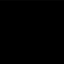MathematicsOpenStudy (anonymous):

Identify the center and radius of each 1. 364+28y+y^2+x^2= -26x 2. x^2+ y^2+ 24x+ 10y+ 160= 0 3. -6x= -x^2+ 32y- 264- y^2 4. -6x+ x^2= 97+10y- y^2OpenStudy (anonymous):

The typical formula for a circle is (x-x1)^2+(y-y1)^2=r^2 where (x1,y1) is the center and r is the radius. So the first thing you want to do is get all of those messy equations into the circle format. Looks like this is a practice for Completing the Square (CTS) #2 looks nice :) x^2+y^2+24x+10y+160=0 first group the x's and the y's together so that we can factor. Might as well subtract 160 from each side too... x^2+24x+y^2+10y=-160 (x^2+24x)+(y^2+10y)=-160 For the x's x^2+24x -> we want to Complete the Square! (CTS) To complete the square, you take 1/2*b (1/2 of the second term's coefficient) and square it! 24x is the second term, 24 is the coefficient, 24=b 1/2 of 24 = 12 12^2 is 144 Then we get (x^2+24x+144)-144 (don't forget to subtract this out... we are trying to "add zero" creatively!) We can factor this into (x+12)^2 -144 For the y's y^2+10y -> CTS! 10y is the second term, 10 is the coefficient 1/2 of 10 = 5 5^2 = 25 Then we get (y^2+10y+25) -25 We can factor this into (y+5)^2 - 25 Putting this all together: (x+12)^2 - 144 + (y+5)^2 - 25 = -160 Simplify by adding 144 and 25 to both sides... (x+12)^2 + (y+5)^2 = 9 so then we know the center is (-12, -5) and the radius is sqrt(9)=3 Remember to use the opposite signs in the parenthesis when finding the center, and to take the square root of the right side to find the radius! Good luck with the other four! HTH!

Latest Questionshayleed: in 800, charlemagne accepted the pope's offer to be crowned holy roman emperor. this act had the practical effect of a.
3 hours ago 0 Replies 0 MedalsGucchi: Object E has a mass of 4,800 kilograms. Object F has a mass of 600 kilograms. The weight of Object F will be ________ times the weight of Object E if both o
5 hours ago 5 Replies 1 Medalprettygirl09: i dont know wat this is but look closly its agirl starring at ze moon..... but te
1 hour ago 5 Replies 1 MedalGucchi: Read the list of substances below: Rubber, Steel, Glass, Iron Which substances ar
5 hours ago 7 Replies 1 MedalGucchi: In a chemical reaction, 36 grams of hydrochloric acid reacts with 40 grams of sodium hydroxide to produce sodium chloride and water.
5 hours ago 5 Replies 1 MedalGucchi: Which state of matter has particles that slide past each other while remaining in
5 hours ago 5 Replies 1 MedalGucchi: Which of the following is true about the mass of an object? It decreases if weight increases.
5 hours ago 6 Replies 1 MedalGucchi: A group of students is investigating how the addition of salt impacts the floatation of an egg in water.
6 hours ago 5 Replies 1 Medalhayleed: Which of the following was an effect of the Crusades on Jews? A. Jewish villages also became targets of the Crusaders.
6 hours ago 0 Replies 0 MedalsGucchi: An electrician cuts a 10-meter-long copper wire having a density 8.9 g/cm3 into 10 equal parts.
6 hours ago 5 Replies 1 Medal